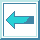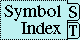#Function FUNCALL

Syntax:

funcall function &rest args => result*

Arguments and Values:

function---a function designator.

args---arguments to the function.

results---the values returned by the function.

Description:

funcall applies function to args. If function is a symbol, it is coerced to a function as if by finding its functional value in the global environment.

Examples:

``` (funcall #'+ 1 2 3) =>  6
(funcall 'car '(1 2 3)) =>  1
(funcall 'position 1 '(1 2 3 2 1) :start 1) =>  4
(cons 1 2) =>  (1 . 2)
(flet ((cons (x y) `(kons ,x ,y)))
(let ((cons (symbol-function '+)))
(funcall #'cons
(funcall 'cons 1 2)
(funcall cons 1 2))))
=>  (KONS (1 . 2) 3)
```

Affected By: None.

Exceptional Situations:

An error of type undefined-function should be signaled if function is a symbol that does not have a global definition as a function or that has a global definition as a macro or a special operator.

Notes:

``` (funcall function arg1 arg2 ...)
==  (apply function arg1 arg2 ... nil)
==  (apply function (list arg1 arg2 ...))
```

The difference between funcall and an ordinary function call is that in the former case the function is obtained by ordinary evaluation of a form, and in the latter case it is obtained by the special interpretation of the function position that normally occurs.

The following X3J13 cleanup issue, not part of the specification, applies to this section: